Learn Polynomials - Start to Finish - FREE. Check - Polynomials Class 10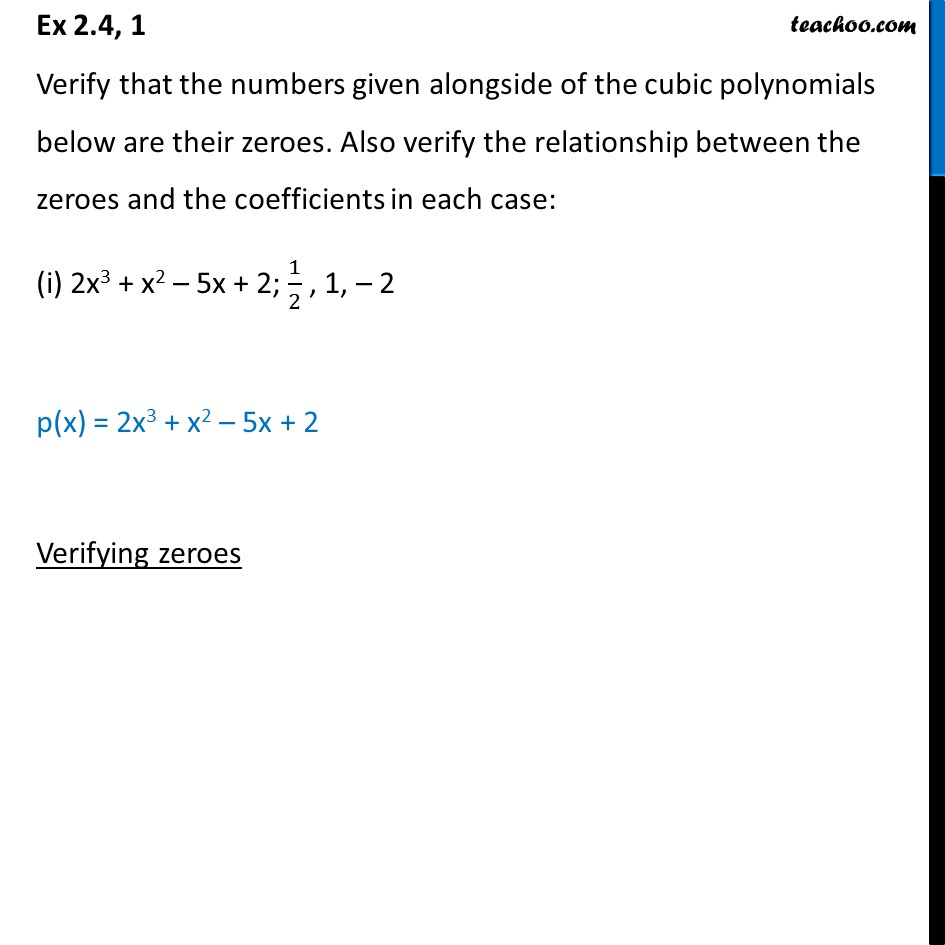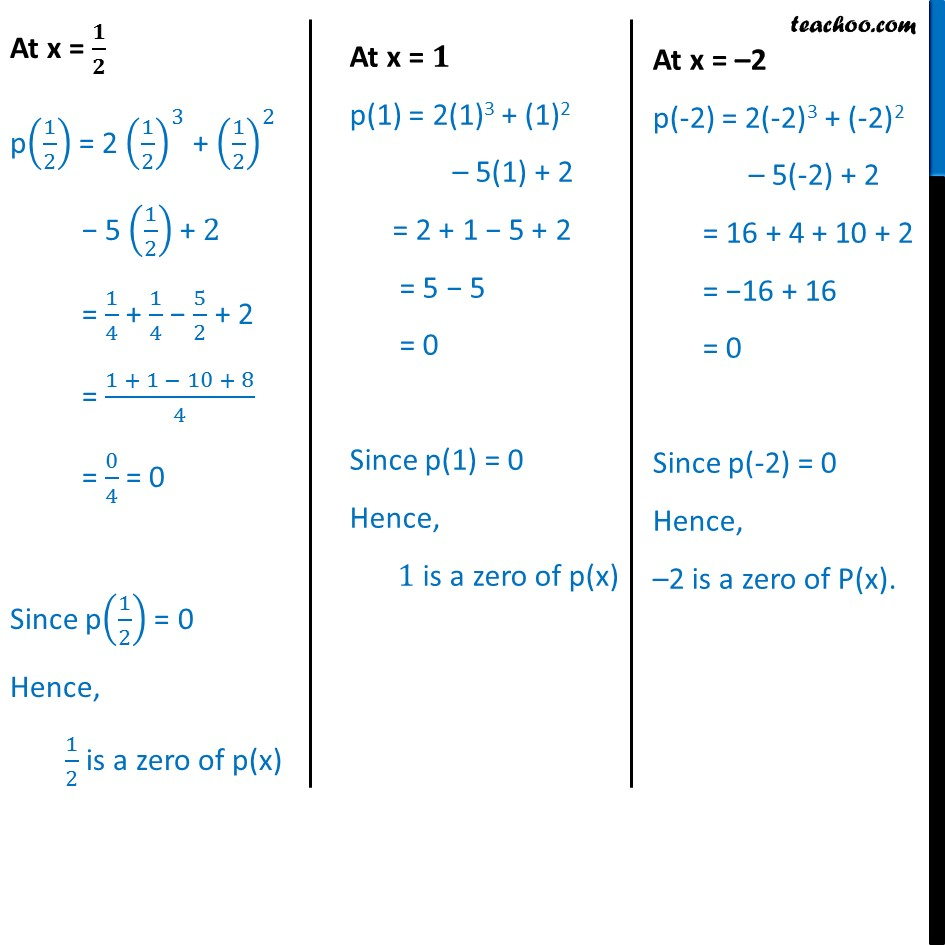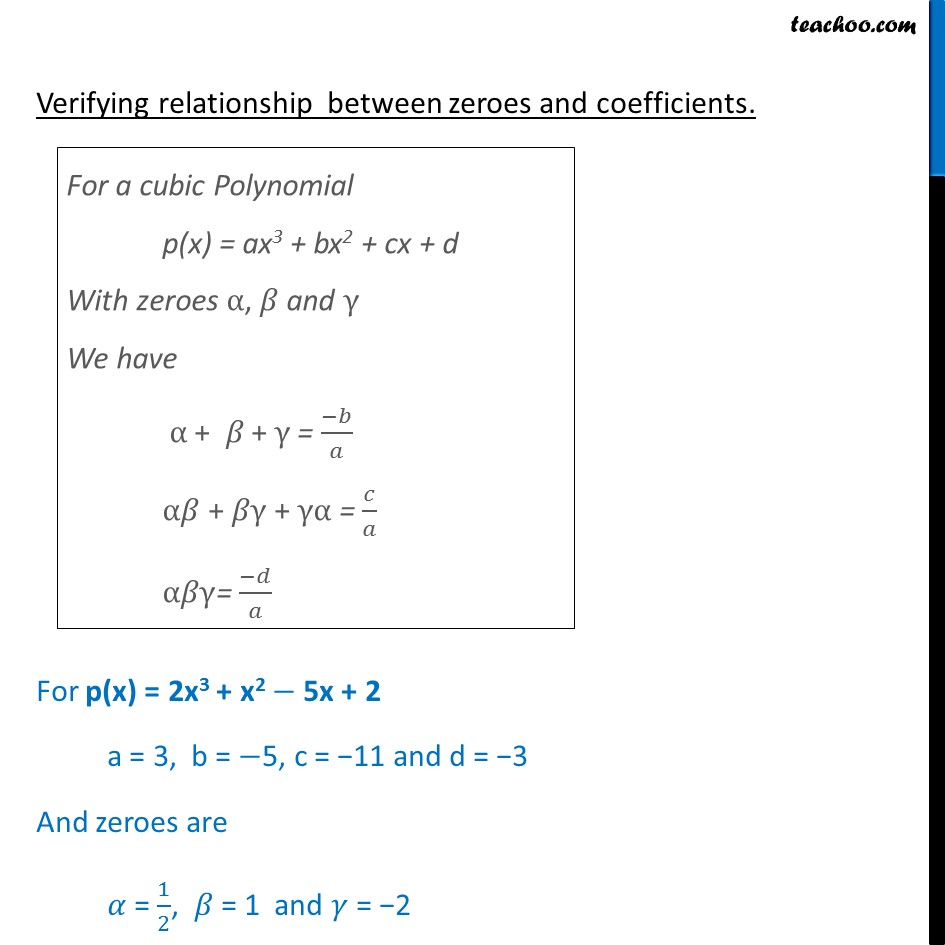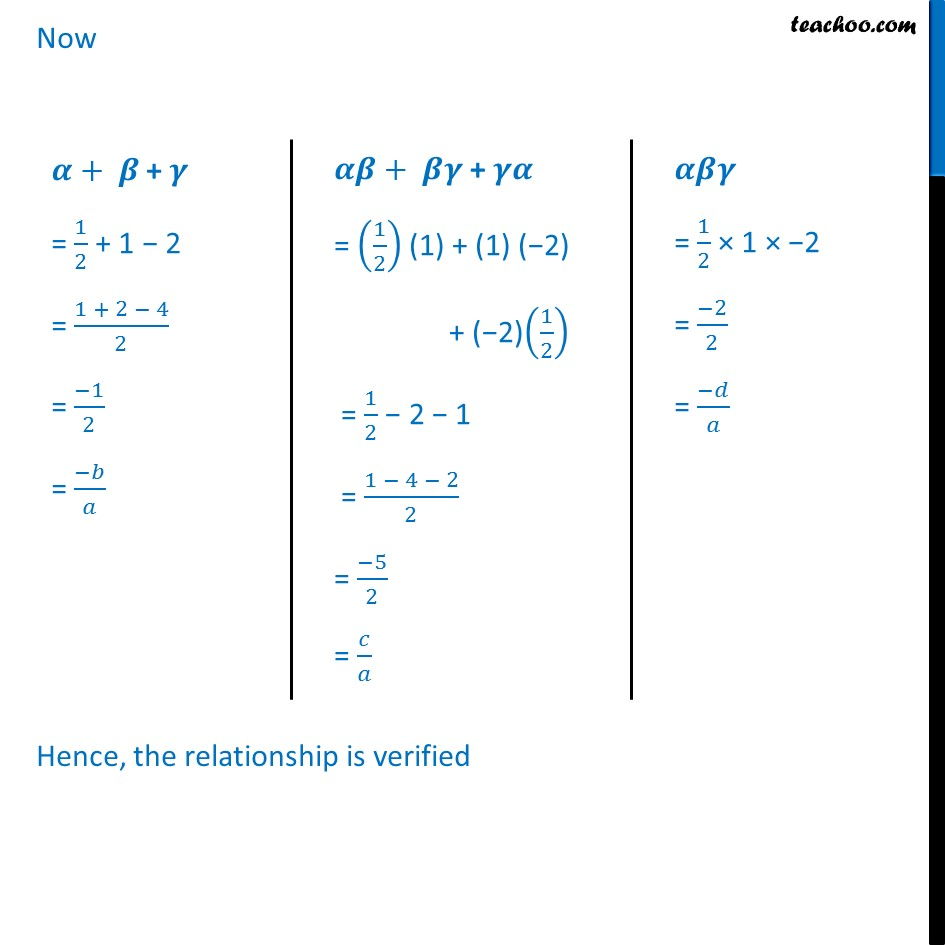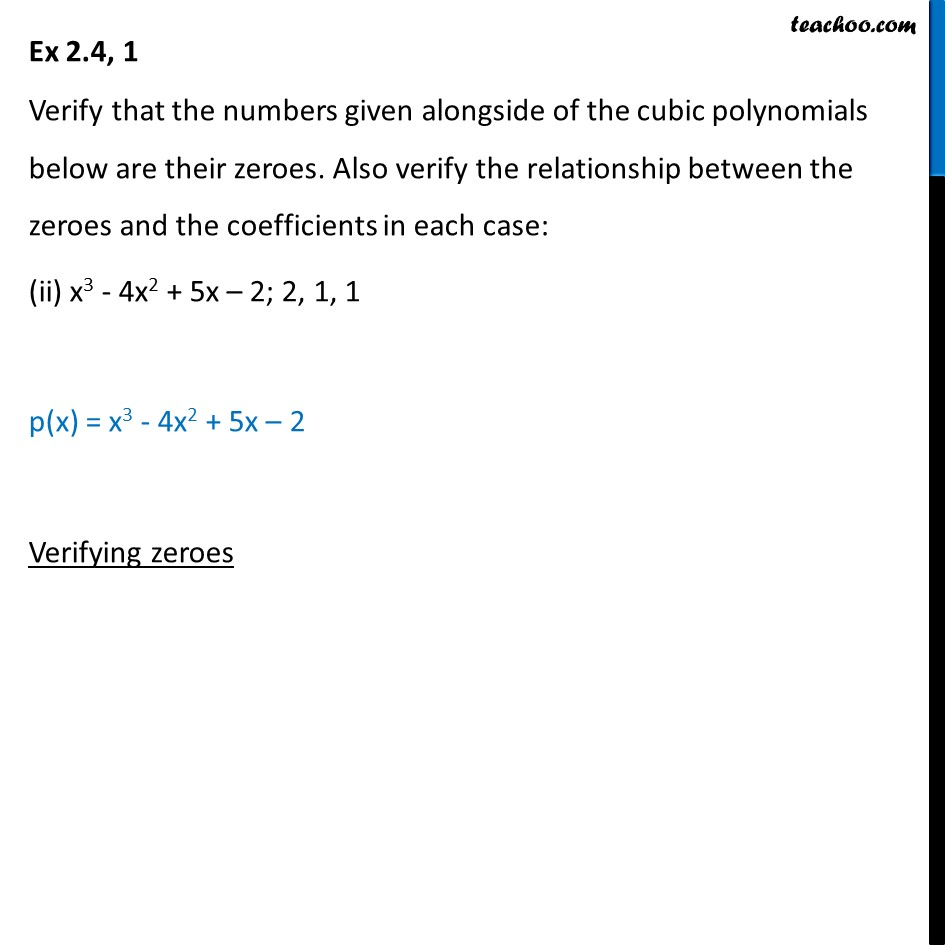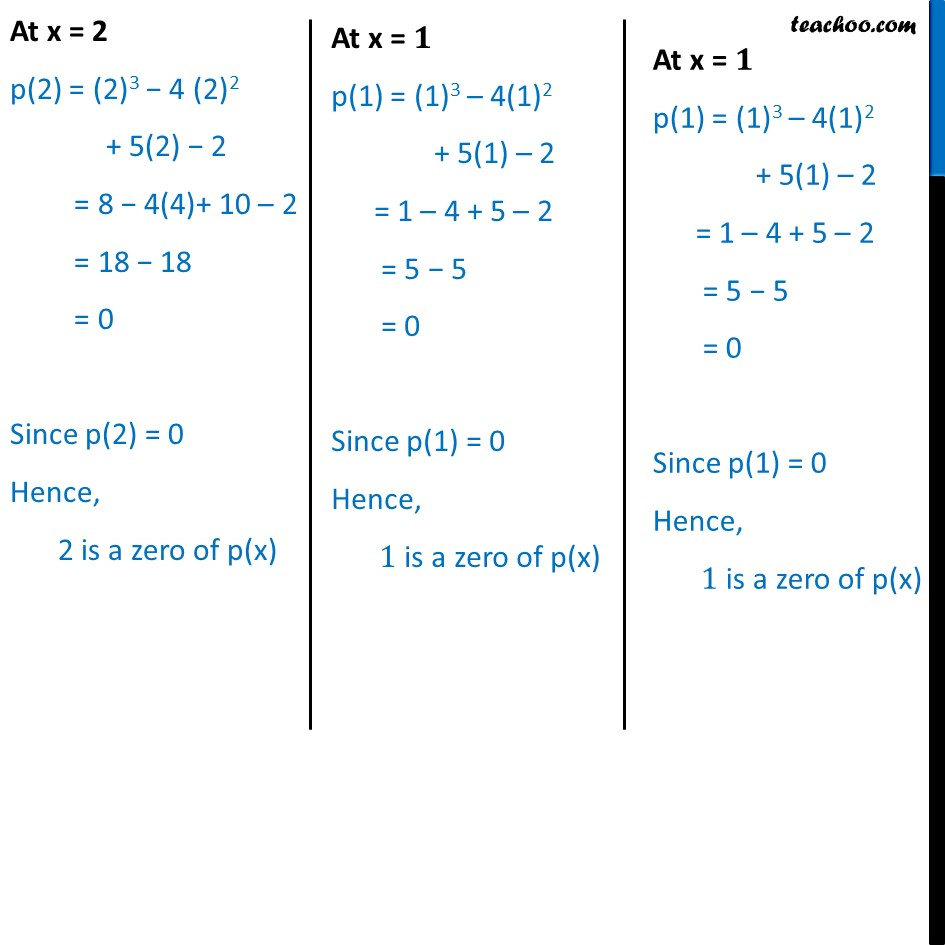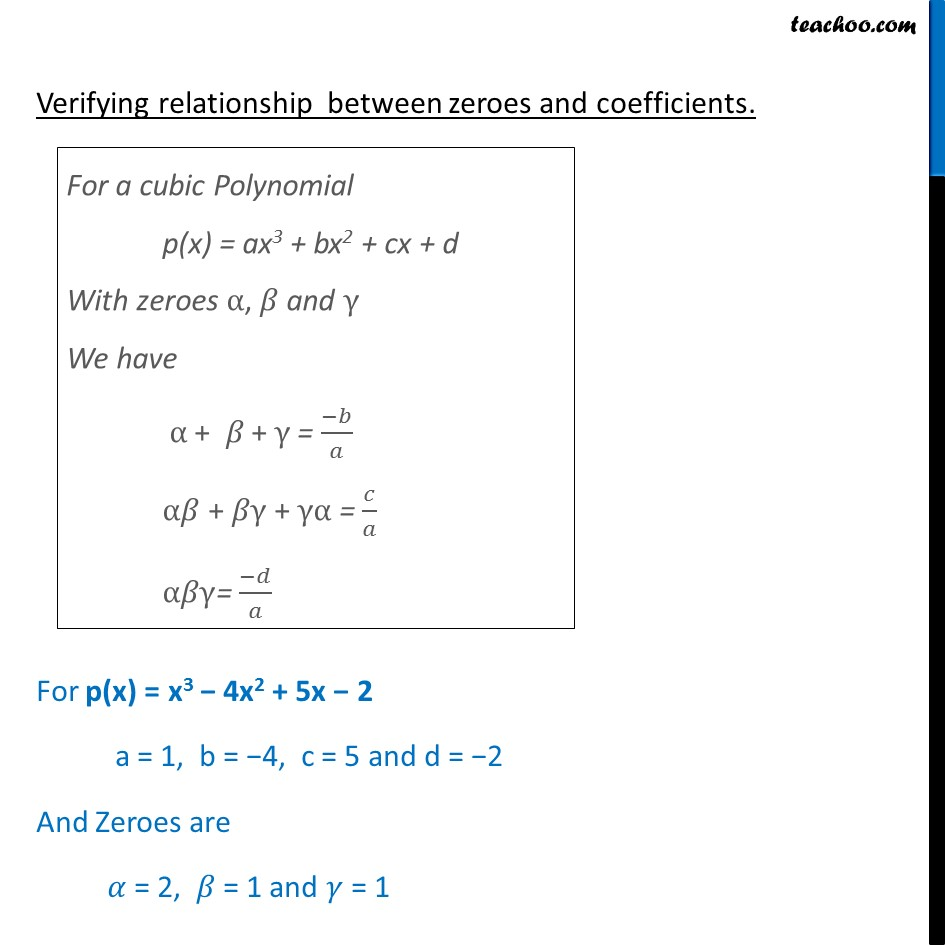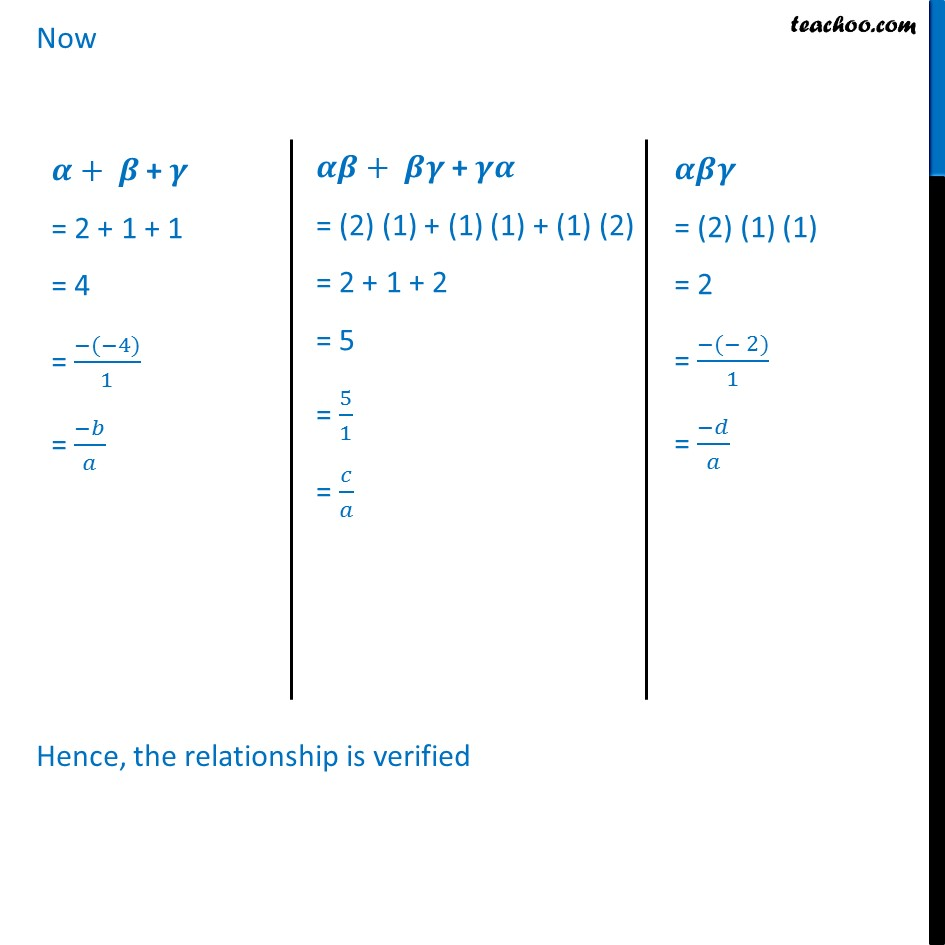1. Chapter 2 Class 10 Polynomials
2. Serial order wise
3. Ex 2.4 (Optional)

Transcript

Ex 2.4, 1 Verify that the numbers given alongside of the cubic polynomials below are their zeroes. Also verify the relationship between the zeroes and the coefficients in each case: (i) 2x3 + x2 5x + 2; 1/2 , 1, 2 p(x) = 2x3 + x2 5x + 2 Verifying zeroes At x = / p(1/2) = 2 (1/2)^3 + (1/2)^2 5 (1/2) + 2 = 1/4 + 1/4 5/2 + 2 = (1 + 1 10 + 8)/4 = 0/4 = 0 Since p(1/2) = 0 Hence, 1/2 is a zero of p(x) At x = p(1) = 2(1)3 + (1)2 5(1) + 2 = 2 + 1 5 + 2 = 5 5 = 0 Since p(1) = 0 Hence, 1 is a zero of p(x) At x = 2 p(-2) = 2(-2)3 + (-2)2 5(-2) + 2 = 16 + 4 + 10 + 2 = 16 + 16 = 0 Since p(-2) = 0 Hence, 2 is a zero of P(x). Verifying relationship between zeroes and coefficients. For a cubic Polynomial p(x) = ax3 + bx2 + cx + d With zeroes , and We have + + = ( )/ " " + + = / " " = ( )/ For p(x) = 2x3 + x2 5x + 2 a = 3, b = 5, c = 11 and d = 3 And zeroes are = 1/2, = 1 and = 2 Now + + = 1/2 + 1 2 = (1 + 2 4)/2 = ( 1)/2 = ( )/ + + = (1/2) (1) + (1) ( 2) + ( 2)(1/2) = 1/2 2 1 = (1 4 2)/2 = ( 5)/2 = / = 1/2 1 2 = ( 2)/2 = ( )/ Hence, the relationship is verified Ex 2.4, 1 Verify that the numbers given alongside of the cubic polynomials below are their zeroes. Also verify the relationship between the zeroes and the coefficients in each case: (ii) x3 - 4x2 + 5x 2; 2, 1, 1 p(x) = x3 - 4x2 + 5x 2 Verifying zeroes At x = 2 p(2) = (2)3 4 (2)2 + 5(2) 2 = 8 4(4)+ 10 2 = 18 18 = 0 Since p(2) = 0 Hence, 2 is a zero of p(x) At x = p(1) = (1)3 4(1)2 + 5(1) 2 = 1 4 + 5 2 = 5 5 = 0 Since p(1) = 0 Hence, 1 is a zero of p(x) At x = p(1) = (1)3 4(1)2 + 5(1) 2 = 1 4 + 5 2 = 5 5 = 0 Since p(1) = 0 Hence, 1 is a zero of p(x) Verifying relationship between zeroes and coefficients. For p(x) = x3 4x2 + 5x 2 a = 1, b = 4, c = 5 and d = 2 And Zeroes are = 2, = 1 and = 1 + + = 2 + 1 + 1 = 4 = ( ( 4))/1 = ( )/ Hence, the relationship is verified

Ex 2.4 (Optional)

Chapter 2 Class 10 Polynomials
Serial order wise